×
Log in to StudySoup
Get Full Access to University Physics - 13 Edition - Chapter 11 - Problem 13e
Join StudySoup for FREE
Get Full Access to University Physics - 13 Edition - Chapter 11 - Problem 13e

Already have an account? Login here
×
Reset your password

# Find the tension T in each cable and the magnitude andISBN: 9780321675460 31

## Solution for problem 13E Chapter 11

University Physics | 13th Edition

• Textbook Solutions
• 2901 Step-by-step solutions solved by professors and subject experts
• Get 24/7 help from StudySoup virtual teaching assistantsUniversity Physics | 13th Edition

4 5 1 348 Reviews
19
4
Problem 13E

Find the tension T in each cable and the magnitude and direction of the force exerted on the strut by the pivot in each of the arrangements in ?Fig. E11.13?. In each case let w be the weight of the suspended crate full of priceless art objects. The strut is uniform and also has weight w. Start each case with a free-body diagram of the strut.

Step-by-Step Solution:

Problem 13E

Find the tension T in each cable and the magnitude and direction of the force exerted on the strut by the pivot in each of the arrangements in Fig. E11.13. In each case let w be the weight of the suspended crate full of priceless art objects. The strut is uniform and also has weight w. Start each case with a free-body diagram of the strut.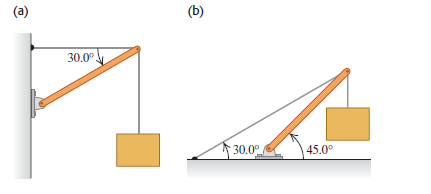Step by Step Solution

Step 1 of 5

(a)

The free body diagram is as follows: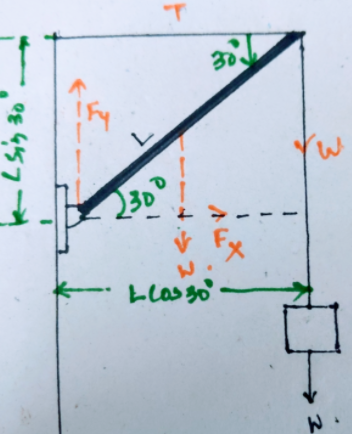The net torque at the pivot is given by: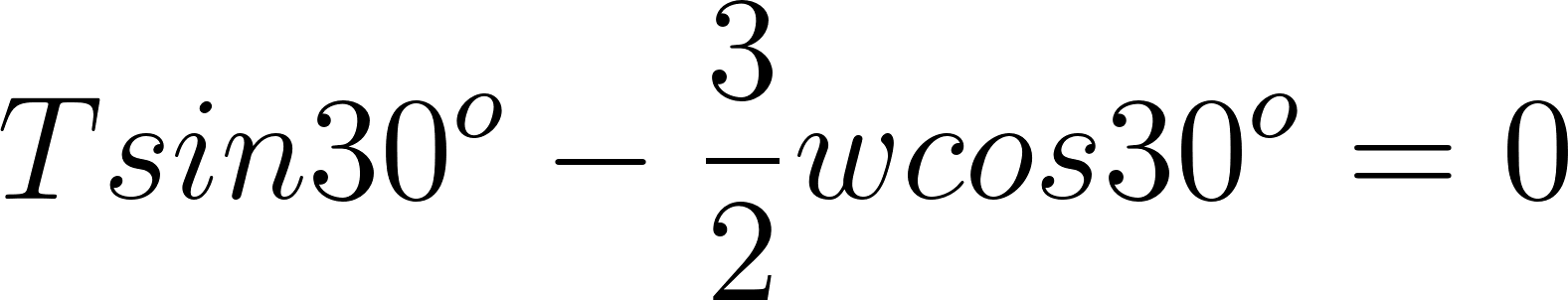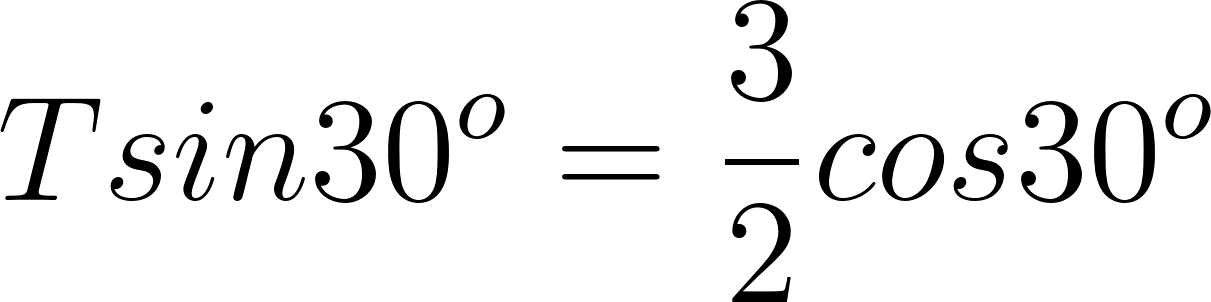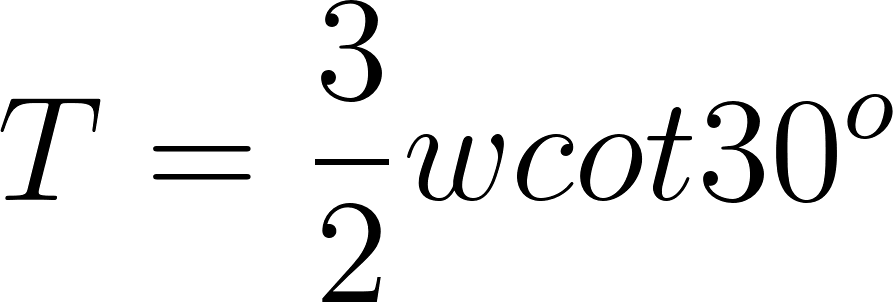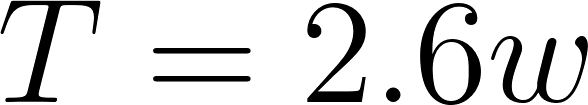Step 2 of 5

Step 3 of 5

##### ISBN: 9780321675460

Since the solution to 13E from 11 chapter was answered, more than 2208 students have viewed the full step-by-step answer. The full step-by-step solution to problem: 13E from chapter: 11 was answered by , our top Physics solution expert on 05/06/17, 06:07PM. This textbook survival guide was created for the textbook: University Physics, edition: 13. University Physics was written by and is associated to the ISBN: 9780321675460. The answer to “Find the tension T in each cable and the magnitude and direction of the force exerted on the strut by the pivot in each of the arrangements in ?Fig. E11.13?. In each case let w be the weight of the suspended crate full of priceless art objects. The strut is uniform and also has weight w. Start each case with a free-body diagram of the strut.” is broken down into a number of easy to follow steps, and 66 words. This full solution covers the following key subjects: strut, weight, Case, free, cable. This expansive textbook survival guide covers 26 chapters, and 2929 solutions.

Unlock Textbook Solution

Enter your email below to unlock your verified solution to:

Find the tension T in each cable and the magnitude and